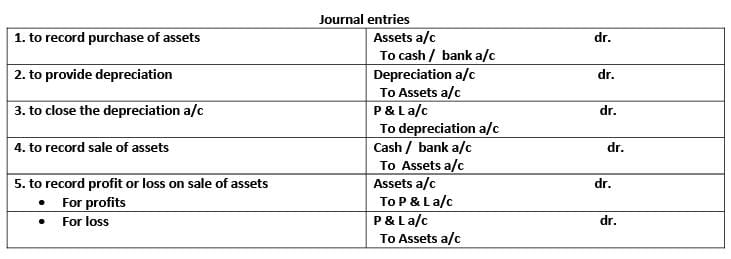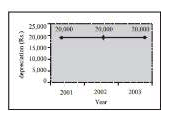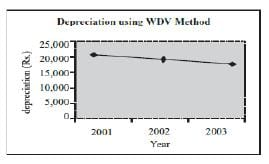# Introduction - Depreciation Notes | Study Crash Course of Accountancy - Class 11 - Commerce

## Commerce: Introduction - Depreciation Notes | Study Crash Course of Accountancy - Class 11 - Commerce

The document Introduction - Depreciation Notes | Study Crash Course of Accountancy - Class 11 - Commerce is a part of the Commerce Course Crash Course of Accountancy - Class 11.
All you need of Commerce at this link: Commerce

Meaning of Depreciation:

Depreciation is a permanent, continuing and gradual decrease in the book value of fixed assets. It is based on the cost of assets consumed in a business and not on its market value.

Features of Depreciation:

1. It is decline in the book value of fixed assets.

2. Such a decline is permanent in nature and once there value is reduced due to depreciation it can’t be restored to its original value.

3. It includes loss of value due to effluxion of time, usage or obsolescence.

4. It is a continuing process.

5. It is a non-cash expense. It does not involve any cash out flow.

Causes of depreciation:

1. Physical wear and tear - When the fixed assets are put to use, the value of such assets may decrease. Such decrease in the value of assets is said to be due to physical wear and tear.

2. With the passage of time - When the assets are exposed to the forces I of nature like weather, winds, rains, etc. the value of such assets may decrease even if they are not put to any use.

3. Changes in economic environment - The value of an asset may decrease due to decrease in the demand of the asset. The demand of the asset may decrease due to technological changes, changes in the habits of consumers etc.

4. Expiration of legal rights - When the use of an asset (e.g., Patents, Leases) is governed by the time bound arrangement, the value of such assets may decrease with the passage of time.

Need, importance & objectives for charging depreciation:

1. To ascertain True Financial Performance - For proper matching of costs with revenues, it is necessary to charge the depreciation (cost) against income (revenue) in each accounting period. Unless the depreciation is charged against income, the financial performance would be overstated. As a result the Income Statement would not present a true and fair view of the financial performance of an accounting entity.

2. To present True and Fair view of the Financial Position - For presenting, a true and fair view of the financial position, it is necessary to charge the depreciation. If the depreciation is not charged, the unexpired cost of the asset concerned would be overstated. As a result, the Position Statement (i.e., the Balance Sheet) would not present a true and fair view of the financial position of an accounting entity.

3. To ascertain the True Cost of Production - For ascertaining the cost of production, it is necessary to charge depreciation as an item of cost of production. If the depreciation on fixed assets is not charged, the cost records, would not present a true and fair view of the cost of production.

4. To comply with Legal Requirements - In case of companies, it is compulsory to charge depreciation on fixed assets before it declares dividend

5. To accumulate Funds for Replacement of Assets - A portion of profits is set aside in the form of depreciation and accumulated each year to provide a definite amount at a certain future date for the specific purpose of replacement of the asset at the end of its useful life.

The amount of depreciation is usually based on the following three factors:

1. Historical cost of a depreciable asset implies the cost incurred on its acquisition, installation, commissioning and for additions to or improvements thereof which are of capital nature.

2. Expected Useful Life of a depreciable asset implies either the period over which a depreciable asset is expected to be used by the enterprise or the number of production or similar units expected to be obtained from the use of theasset by the enterprise.

3. Estimated Residual Value of a depreciable asset implies the value expected to be realised on its sale or exchange on the expiry of its useful life.

Depreciation And Other Related Concepts:

(i) Depreciation: Depreciation means decrease in the book value of tangible fixed assets due to their use in business, efflux (passage) of time or obsolescence.

(ii) Depletion: The term Rs.depletion’ is used in relation of natural resources like quarries, oil wells, mines, etc. When natural resources are extracted or exhausted their stock value is reduced. This reduction is termed as depletion.

(iii) Amortisation: Amortisation means writing off of intangible fixed assets like goodwill, patents, trademarks, copyright, etc.

(iv) Obsolescence: It refers to decline in the economic value of the asset due to innovation or improved technique, change in taste or fashion or inadequacy of the existing asset due to improved demandMethods for depreciation:

Straight Line Method-

Under the Straight Line Method, a fixed and equal amount of depreciation, calculated at a fixed percentage on the original cost of a Fixed Depreciable Asset is written off during each accounting period over the expected useful life of the asset.

It is also known as original cost method / equal instalment method / fixed instalment method

Formula for depreciation-

• Depreciation =  total cost – scrap value

Estimated life

• Depreciation = total cost x  rate

100

• Rate of Depreciation = Amount of Depreciation x 100

Original Cost

For example - the original cost of the asset is Rs. 2,50,000, The useful life of the asset is 10 years and net residual value is estimated to be Rs. 50,000. Now, the amount of depreciation to be charged every year will be computed as given below –

depreciation = total cost – scrap value

Estimated life

= 250000– 50000   = 20,000

10Merits of Straigth Line Method:

1. It is easy to understand.

2. It is easy to calculate the amount and rate of depreciation.

3. Under this method, the book value of the asset becomes zero or equal to its scrap value at the expiry of its useful life.

Demerits of Straigth Line Method:

1. The total charge (i.e., Depreciation plus Repairs and Renewals) in later years is more as compared to that in earlier years since, the amount of repairs and renewals goes on increasing as the asset grows older whereas the amount of depreciation remains constant year after year.

2. It does not take into consideration the interest on the capital invested in the asset.

3. It does not provide for the replacement of the asset on the expiry of its useful life.

SLM is suitable for those assets in relation to which

(a) repair charges are less, and

(b) the possibility of obsolescence is less. This method is suitable for Furniture, Patent, Copyright, Trademark, Lease etc.

'Written Down Value Method' of Depreciation (WDV):

Under the Written Down Value method, depreciation calculated at a fixed percentage on the Original Cost (in the first year) and on the Written Down Value, (in subsequent years) of a Fixed Depreciable Asset is written off during each accounting period over the expected useful life of the asset. Under this method, the rate of depreciation remains constant year after year whereas the amount of depreciation goes on decreasing It is also known as diminishing balance method / reducing balance method For example, the original cost of the asset is Rs. 2,00,000 and depreciation is charged @ 10% p.a. at written down value, then the amount of depreciation will be computed as follows:(i) Depreciation (I year) Rs. 20,00,000 × 10   =  20,000

100

(ii) Written down value = Rs. 2,00,000 – 20,000 = Rs.1,80,000 (at the end of the I year) (iii) Depreciation (II year) = Rs. 18,000 Rs. 1,80,000 × 10  =  18,000

100

(iv) Written down value = Rs. 1,80,000 – Rs.18,000 = 1,62,000 (at the end of the II year) (v) Depreciation (III year) = Rs. 16,200 Rs. 1,62,000 × 10   =  16,200

10

(vi) Written down value = Rs. 1,62,000 – Rs. 16,200 = Rs. 1,45,800

Merits of WDV Method:

1. The total charge (i.e., Depreciation plus Repairs Renewals) remains almost uniform year after year, since in earlier years the amount of Depreciation I is more and the amount of Repairs and Renewals is less, whereas in later years the amount of Depreciation is less and the amount of Repairs & Renewals is more.

2. This method is logical in the sense that as the asset grows older, the amount of depreciation also goes on decreasing.

Demerits of WDV Method:

1. It is difficult to calculate the rate of depreciation.

2. It does not take into consideration the interest on capital invested in the asset.

3. It does not provide for the replacement of the asset on the expiry of its useful life.

4. It takes a very long time to write an asset down to its break-up value, unless a very high rate is used.

Comparison of straight line and written down value method:

 Basis of Difference Straight Line Method Written Down Value Method 1. Basis of charging depreciation Original cost Book Value (I.e. original cost less depreciation charged till date) 2. Annual depreciation  charge Fixed (Constant) year Declines year after year 3. Total charge against profit and loss account In respect of depreciation and repairs Unequal year alter year. It Increases In later years. Almost equal every year. 4. Recognition by Income tax law Not recognised Recognised 5. Sultabllty It is suitable for assets in which repair charges are less. the possibility of and obsolescence Is low scrap value depends upon the time period Involved. It is suitable for assets. which are affected by technological changes and require more repair expenses with passage of time.
The document Introduction - Depreciation Notes | Study Crash Course of Accountancy - Class 11 - Commerce is a part of the Commerce Course Crash Course of Accountancy - Class 11.
All you need of Commerce at this link: CommerceUse Code STAYHOME200 and get INR 200 additional OFF

## Crash Course of Accountancy - Class 11

67 docs|53 tests

Track your progress, build streaks, highlight & save important lessons and more!

,

,

,

,

,

,

,

,

,

,

,

,

,

,

,

,

,

,

,

,

,

;